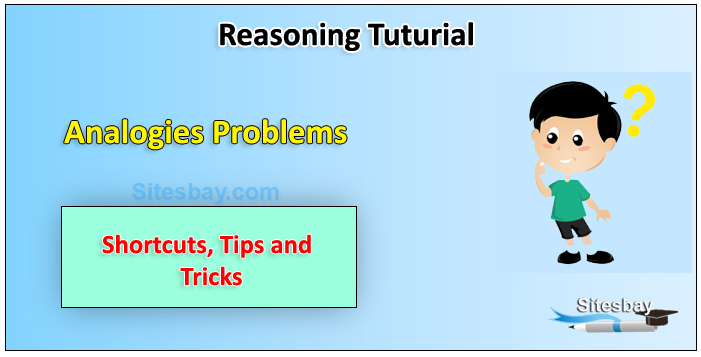# Analogies Tips and Tricks

## Analogies Problem Tips and Tricks

Analogies are often used in assessments and intelligence measurements. Analogy means similarity. In this type of questions, two objects related in some way are given and third object is also given with four or five alternatives. You have to find out which one of the alternatives bears the same relation with the third objects as first and second objects are related.### Types of Analogies

In reasoning, generally we come across two types of analogies: Alphabet analogy and Numbers analogy. Number Analogy covers two types of questions:

• Choose a similar pair as the given pair in the question on the basis of the relation between the numbers pair given in the question.
• Choose a number that is similar to a group of numbers on the basis of certain similar properties that they possess.

Question 1: ABC : ZYX :: CBA : ?

• (A) XYZ
• (B) BCA
• (C) YZX
• (D) ZXY

Explanation: CBA is the reverse of ABC similarly XYZ is the reverse of ZYX.

Question 2: 4 : 18 :: 6 : ?

• A - 32
• B - 38
• C - 11
• D - 37

Explanation: As, (4)2 + 2 =18, Similarly, (6)2 + 2 = 38..

Question 3: Calf : Piglet :: Shed : ?

• A - Prison
• B - Nest
• C - Pigsty
• D - Den

Explanation: Calf is young one of the cow and piglet is the young of Pig. Shed is the dwelling place of cow. Similarly Pigsty is the dwelling place of pig.

Question 4: Flow : River :: Stagnant : ?

• A - Rain
• B - Stream
• C - Pool
• D - Canal

Explanation: As Water of a River flows similarly water of Pool is Stagnant.